# Hall Current Effects on Binary Mixture Flow of Oldroyd-B Fluid through a Porous Channel

Hall Current Effects on Binary Mixture Flow of Oldroyd-B Fluid through a Porous Channel

Debasish DeyArdhendu Sekhar Khound

Department of Mathematics, Dibrugarh University, Dibrugarh- 786004, India

Corresponding Author Email:
debasish41092@gmail.com
Page:
687-693
|
DOI:
https://doi.org/10.18280/ijht.340419
|
Accepted:
|
Published:
31 December 2016
| Citation

OPEN ACCESS

Abstract:

Free convective binary mixture flow of visco-elastic fluid through a porous channel in presence of Hall effects, radiation and first order chemical reaction has been considered. A magnetic field of uniform strength has been applied along the transverse direction to the channel. The visco-elastic fluid flow is governed by Oldroyd fluid-B model. Two important rheological parameters involved in the constitutive equation are relaxation parameter and retardation parameter. In the mixture, one of the components is assumed to be rarer lighter. The temperature and concentration of the fluid at one surface is assumed to be constant but temperature and concentration of the fluid at the second surface are assumed to be oscillating about a constant temperature and concentration respectively. The governing equations of momentum, temperature and concentration are solved analytically by using separation of variable technique. Results are discussed graphically for various values of flow parameters involved in the solution.

Keywords:

Relaxation and retardation, Oldroyd-B fluid model, Free convection, Separation of variable, Shearing stress.

1. Introduction

Mechanism of relaxation and retardation parameters in the Oldroyd fluid model has attracted many researchers as it can study the visco-elastic fluid motion in more generalized way. The constitutive equation of Oldroyd fluid model (Oldroyd , ) is given by

$\sigma_{i j}=-p \delta_{i j}+\tau_{i j} \&\left(1+\lambda_{1} \frac{d}{d t}\right) \tau_{i j}=2 \mu\left(1+\lambda_{2} \frac{d}{d t}\right) e_{i j}$(1.1)

Dey and Dey and Khound have investigated the effects of relaxation and retardation of visco-elasticity on governing fluid motion. Nigam and Singh  have derived the asymptotic solutions of the energy equation of heat-transfer problem of fluid flow between two infinite parallel plates. Soundalgekar and Bhat  have investigated oscillatory channel flow and heat transfer. Hydro-magnetic Couette flow with heat transfer and Hall effects has been investigated by Soundalgekar et al. , Saoundalgekar and Uplekar  and Hossain and Rashid . Raptis et al. , Raptis and Perdikis , Hassanien and Mansour  and Aldoss et al.   have analysed hydro-magnetic flow problem through a porous medium. Vajravelu  have obtained an exact periodic solution of a hydromagnetic flow in a horizontal channel considering hydro-magnetic and hydrodynamic cases. Attia and Kotb  have discussed numerically the problem of steady flow bounded by two parallel infinite insulated horizontal plates and the heat transfer.  Hall current effects of unsteady Hartmann flow between two parallel porous plate of Newtonian and visco-elastic fluid have been analysed by Attia( & ). Closed form solution of heat and mass transfer problem in elastico-viscous fluid past an impulsively started infinite vertical plate with Hall effect has been obtained by Choudhury and Jha. Effect of oscillatory motion of visco-elastic fluid over an infinite stretching sheetthrough porous media in the presence of magnetic field with applied suction has been studied by Rajagopal et al. . Radiative heat transfer in MHD oscillatory flow through porous medium bounded by two vertical porous plates has been analysed by Singh and Garg . Singh  have investigated visco-elastic mixed convective MHD oscillatory flow through a porous medium filled in a vertical channel. Exact solution of MHD mixed convection periodic flow in a rotating vertical channel with heat radiation has been derived by Singh . Singh and Pathak  have analysed the Effects of slip conditions and Hall current on an oscillatory convective flow in a rotating vertical porous channel with thermal radiation. Seth et al. (, ) have studied the effects of Hall current in presence of ramped temperature. Ahmed et al.  have investigated the effects of Hall current on MHD mass transfer flow in a rotating system.

In various science and technology problems, separation process of binary mixture components has been used. In chemical industry, problem of visco-elastic fluid flow with heat and mass transfer is often used to study polymer solution mixed with various organic compounds. Examples of multiple component electrically conducting fluids are molten fluid in earth’s crust, crude oil in petroleum etc . In our study, we have assumed a binary mixture of visco-elastic fluid, where one of component is present in extremely small proportion.

The objective of this problem is to investigate the problem of oscillatory binary mixture flow of visco-elastic fluid with relaxation and retardation through a porous channel. Singh et al  have studied the hall current effects of visco-elastic fluid flow governed by second order fluid model though a porous medium with radiative heat transfer. The other physical properties like free convection (heat and mass transfer due to density differences) and first order chemical reaction also have been considered in this flow problem.

2. Mathematical Formulation

An unsteady binary mixture flow of visco-elastic fluid characterized by Oldroyd model past a porous channel has been investigated. The channel is bounded by two infinite vertical plates separated by distance d. Here x-axis is taken along the length of the plates and z-axis is taken perpendicular to it. To stable the system, a magnetic field is applied along the transverse direction to the surface. Application of transverse magnetic field generates Lorentz force and Hall current. In the mixture, let C1 and C2 be the concentrations of lighter and heavier components respectively and C2= 1- C1. The motion of binary mixture is similar to normal fluid flow with velocity $u^{\prime}=\frac{u_{1} \rho_{1}+u_{1} \rho_{1}}{\rho_{1}+\rho_{2}}$ and density $\rho=\rho_{1}+\rho_{2}$, where $\rho_{1}, \quad \rho_{2}, u_{1} \& u_{2}$ are densities and velocities of rarer and heavier components respectively.

## 1.png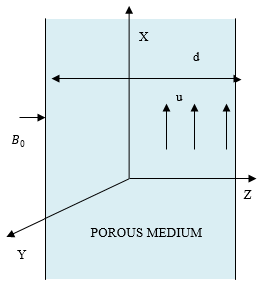Figure 1. Geometry of the problem

Momentum equation:

\begin{aligned}\left(1+\lambda_{1} \frac{\partial}{\partial t^{\prime}}\right) \frac{\partial u^{\prime}}{\partial t^{\prime}} &=-\frac{1}{\rho}\left(1+\lambda_{1} \frac{\partial}{\partial t^{\prime}}\right) \frac{\partial p *}{\partial x^{\prime}}+\frac{1}{\rho} \frac{\partial}{\partial z^{\prime}}\left[\mu\left(1+\lambda_{2} \frac{\partial}{\partial t^{\prime}}\right) \frac{\partial u^{\prime}}{\partial z^{\prime}}\right] \\ &+\left(1+\lambda_{1} \frac{\partial}{\partial t^{\prime}}\right)\left[\sigma B_{0}^{2}\left(\frac{m v^{\prime}-u^{\prime}}{1+m^{2}}\right)-\frac{v u^{\prime}}{k_{p}^{\prime}}+g \beta\left(T^{\prime}-T_{0}\right)+g \beta^{*}\left(C^{\prime}-C_{0}\right)\right] \end{aligned}(2.1)

$\left(1+\lambda_{1} \frac{\partial}{\partial t^{\prime}}\right) \frac{\partial v^{\prime}}{\partial t^{\prime}}=-\frac{1}{\rho}\left(1+\lambda_{1} \frac{\partial}{\partial t^{\prime}}\right) \frac{\partial p *}{\partial y^{\prime}}+\frac{1}{\rho} \frac{\partial}{\partial z^{\prime}}\left[\mu\left(1+\lambda_{2} \frac{\partial}{\partial t^{\prime}}\right) \frac{\partial v^{\prime}}{\partial z^{\prime}}\right]+\left(1+\lambda_{1} \frac{\partial}{\partial t^{\prime}}\right)\left[-\sigma B_{0}^{2}\left(\frac{m u^{\prime}+v^{\prime}}{1+m^{2}}\right)-\frac{v v^{\prime}}{k^{\prime} p}\right]$(2.2)

Energy equation:

$\rho C_{p} \frac{\partial T^{\prime}}{\partial t \prime}=k \frac{\partial^{2} T^{\prime}}{\partial z^{\prime 2}}-\frac{\partial q^{\prime}}{\partial z^{\prime}}$(2.3)

For a optically thin fluid, the radiative heat transfer (Cogley et al. ) is given by,$\frac{\partial q^{\prime}}{\partial z^{\prime}}=4 \alpha^{2}\left(T^{\prime}-T_{0}\right),$ where, $\alpha^{2}=\int_{0}^{\infty} K_{\lambda w} \frac{d e_{\lambda^{\prime} h}}{d T} d \lambda^{\prime}, K_{\lambda w}$ is absorption coefficient and $e_{\lambda^{\prime} h}$ is Plank’s function.

Neglecting the pressure diffusion co-efficient and thermal diffusion coefficient, we get the energy equation for species concentration ($C_{1}=C^{\prime}$) in presence of first order chemical reaction (rate of reaction is proportional to concentration) as follows :

$\frac{\partial C^{\prime}}{\partial t^{\prime}}=D \frac{\partial^{2} C^{\prime}}{\partial z^{\prime 2}}-k_{1}\left(C^{\prime}-C_{0}\right)$(2.4)

Here, $p *=p^{\prime}-p_{s}$ pressure difference, $p^{\prime}$ fluid pressure and  $\mathcal{P}_{S}$ fluid pressure at static case.

The corresponding boundary conditions are as follows:

\begin{aligned} u^{\prime} &=0 ; v^{\prime}=0 ; T^{\prime}=T_{0} ; C^{\prime}=C_{0} ; z^{\prime}=-\frac{d}{2} \\ u^{\prime} &=0 ; v^{\prime}=0 ; T^{\prime}=T_{0}+\left(T_{w}-T_{0}\right) e^{\omega^{\prime} t^{\prime}} ; \\ C^{\prime} &=C_{0}+\left(C_{w}-C_{0}\right) e^{\omega^{\prime} t^{\prime}} ; z^{\prime}=\frac{d}{2} \end{aligned}(2.5)

3. Method of Solution

The following non-dimensional quantities have been used into the equations from (2.1) to (2.4) to make them dimensionless.

$x=\frac{x^{\prime}}{d} ; y=\frac{y^{\prime}}{d} ; z=\frac{z^{\prime}}{d} ; u=\frac{u^{\prime}}{U} ; v=\frac{v^{\prime}}{U} ; t=\frac{t^{\prime} U}{d}$

$p=\frac{p *}{\rho U^{2}} ; \omega=\frac{\omega^{\prime} d}{U} ; T=\frac{T^{\prime}-T_{0}}{T_{w}-T_{0}} ; C=\frac{C^{\prime}-C_{0}}{C_{w}-C_{0}} ; a=\frac{\lambda_{1} U}{d}$

$b=\frac{\lambda_{2} U}{d} ; M=\frac{\sigma B_{0}^{2} d^{2}}{v}$

$G_{r}=\frac{g \beta d^{2}\left(T_{w}-T_{0}\right)}{v U} ; G_{m}=\frac{g \beta^{*} d^{2}\left(C_{w}-C_{0}\right)}{v U} ; h=\frac{d^{2} k_{1}}{D}$.

$P e=\frac{\rho C_{p} U d}{k} ; N=\frac{2 \alpha d}{\sqrt{k}} ; h=\frac{d^{2} k}{D} ; k_{p}=\frac{k_{p}^{\prime}}{d} ; m=\omega_{e} \tau_{e}$

The dimensionless equations are as follows:

$\operatorname{Re}\left(\frac{\partial u}{\partial t}+a \frac{\partial^{2} u}{\partial t^{2}}\right)=-\operatorname{Re}\left(1+a \frac{\partial}{\partial t}\right) \frac{\partial p}{\partial x}+\frac{\partial^{2} u}{\partial z^{2}}+b \frac{\partial^{3} u}{\partial z^{2} \partial t}+\left(1+a \frac{\partial}{\partial t}\right)\left[M\left(\frac{m v-u}{1+m^{2}}\right)-\frac{u}{k_{p}}+G_{r} T+G_{m} C\right]$(3.1)

$\left(\frac{\partial v}{\partial t}+a \frac{\partial^{2} v}{\partial t^{2}}\right)=-\operatorname{Re}\left(1+a \frac{\partial}{\partial t}\right) \frac{\partial p}{\partial y}+\frac{\partial^{2} v}{\partial z^{2}}+b \frac{\partial^{3} v}{\partial z^{2} \partial t}+\left(1+a \frac{\partial}{\partial t}\right)\left[-M\left(\frac{m u+v}{1+m^{2}}\right)-\frac{v}{k_{p}}\right]$(3.2)

$P e \frac{\partial T}{\partial t}=\frac{\partial^{2} T}{\partial z^{2}}-N^{2} T$(3.3)

$S c \frac{\partial C}{\partial t}=\frac{\partial^{2} C}{\partial z^{2}}-h C$(3.4)

Equations (3.1) and (3.2) can be combined into a single differential equation by assuming $F=u+i v$ and we get,

$\operatorname{Re}\left(1+a \frac{\partial}{\partial t}\right)\left(\frac{\partial F}{\partial t}+\frac{\partial p}{\partial x}+i \frac{\partial p}{\partial y}\right)=\frac{\partial^{2} F}{\partial z^{2}}+b \frac{\partial^{3} F}{\partial z^{2} \partial t}+\left(1+a \frac{\partial}{\partial t}\right)\left[\frac{-M F(1+i m)}{1+m^{2}}-\frac{F}{k_{p}}+G_{r} T+G_{m} C\right]$(3.5)

Following (), the pressure gradient of the oscillatory flow is taken as $\frac{\partial p}{\partial x}=-A e^{i \omega t}, \frac{\partial p}{\partial y}=0$

To solve the above equations from (3.3) to (3.5) the following boundary conditions are used

$T=C=F=0 ; z=-\frac{1}{2} \&$

$T=C=e^{i \omega t}, F=0 ; z=\frac{1}{2}$(3.6)

To solve the above equations separation of variable method is used and the complex form of the solution corresponding to the boundary are taken as

$T=T_{1}(z) e^{i \omega t}, C=C_{1}(z) e^{i \omega t} \& F=f_{0}(z) e^{i \omega t}$(3.7)

Using (3.7) into the equations and equating the like terms, we get

$T_{1}^{\prime \prime}-\left(N^{2}+i \omega P e\right) T_{1}=0$(3.8)

$C_{1}^{\prime \prime}-(h+i \omega S c) C_{1}=0$(3.9)

$f_{0}^{\prime \prime}+\left(A_{7}+i A_{8}\right) f_{0} =-\left(A_{9}+i A_{10}\right)\left[G_{r}\left\{B_{1} e^{\left(A_{1} Z+i A_{2} Z\right)}+B_{2} e^{\left(-A_{1} Z-i A_{2} Z\right)}\right\}+G_{M}\left\{B_{3} e^{\left(A_{3} Z+i A_{4} Z\right)}+B_{4} e^{\left(-A_{3} Z-i A_{4}Z\right)}\right\}\right] +\left(A_{11}+i A_{12}\right) R e$(3.10)

The relevant boundary conditions are as follows:

$T_{1}=C_{1}=f_{0}=0 ; z=-\frac{1}{2} \& T_{1}=C_{1}=1, f_{0}=0 ; z=\frac{1}{2}$(3.11)

Solution of the equations (3.8) to (3.10) corresponding to boundary conditions (3.11) are obtained but not presented here for the sake of brevity.

4. Results and Discussions

Figure 2 to 8 represent the velocity profiles against the displacement variable for primary and secondary flows for various values of flow parameters involved in the solution. The figures enable the fact that the effect of flow parameters is more prominent in the centre of the channel. It is seen that during the growth of relaxation parameter, fluid flows (both primary and secondary) have experience an increasing speed as it can be interpreted that during the growth of relaxation parameter (figure 2), stress relaxes more rapidly, and more energy can be stored, as a consequence speed increases. Retardation parameter (figure 3) is connected with creeping motion of visco-elastic fluid and increasing values of retardation parameter accelerates the fluid motion, as physically it is interpreted as the growth of retardation parameter reduces the creepiness of fluid flows (primary and secondary).

Application of transverse magnetic field generates  a  force field known as Lorentz force, the combination of Lorentz force and viscosity makes the system thicker and as a result speed slows down. This physical phenomenon is clearly seen in our result [figure 4]. During the growth of M (magnetic parameter) by 27.27% (from M=2.2 to 2.8), there is a fall in magnitude of velocity by 91% (approximately) at the central portion of the channel. The Hall current parameter also has a negative impact on the motion (figure 5) as it decelerates the fluid motion by 99.36% (approximately) at the central portion of channel during the enhancement of m by 60% only. Thus the influence of m (Hall current parameter) on fluid motion is greater than M (magnetic parameter).

Another force which guides the fluid motion is the pressure gradient and its effect on fluid motion is shown by figure 6 and 7 and it is seen that as magnitude of pressure gradient increases by 300%, maximum increment of speed of primary flow is observed as 62.5% (approximately) and speed of secondary fluid motion is increased by 66.67%.

Formation of viscous drag of governing fluid motion at the surface is very useful in aerodynamics as the wings of aeroplane are constructed in such a manner that shearing stress should be less. In our study we have shown the effects (figure 8 to 12) of visco-elastic parameters, magnetic parameter, Hall current parameter, pressure gradient and chemical reaction on shearing stress or viscous drag at z= -1/2 in the time period [0, 20]. The oscillating nature of shearing stress with respect to time is clearly seen from the figures. Figures 8 and 9 depict the nature of shearing stress formed by primary and secondary fluid motion during Newtonian fluid (a=0, b=0) and visco-elastic fluid (a =1.35, b=3.5). It is noticed that during the motion of visco-elastic fluid, the amplitude of variation of shearing stress is increased by 39% (approximately) in compare Newtonian fluid. It is due to the presence creepiness of friction and resistance forces in visco-elastic fluid motion. During the oscillatory nature of shearing stress, phase difference is seen between Newtonian fluid and visco-elastic fluid.

Effects of pressure gradient on shearing stresses are represented by figure 10 and it tells that during the growth in magnitude of pressure gradient (A) by 300% there is a fall by 19% (approximately) in shearing stress it shows the power of pressure gradient is dominant over frictional force. A fall in shearing stress by 8% and 25% respectively have been noticed during the growth of magnetic parameter by 27.27% (figure 11) and Hall current parameter by 60% (figure 12).

5. Conclusions

Some of the important points from the above study are highlighted as below:

• To control the fluid motion, magnetic parameter or Hall current parameter may be increased.
• Speed of fluid motion experiences an increasing trend with pressure gradient.
• Growth of pressure gradient, magnetic parameter, Hall current parameter and chemical reaction parameter helps to reduce the strength of shearing stress at the surface.
• Amplitude of the shearing stress of visco-elastic fluid motion is higher than the shearing stress formed due to the motion of Newtonian fluid.
6. Graphs

## 2.png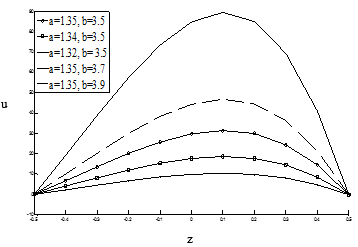Figure 2. Primary velocity against z for $\omega$=0.01, M=2, Re=0.3, Pe=8, N=8, $G_{m}$=4, $G_{r}$=7, h=0.5, Sc=2, $k_{p}$=0.2, m=1,  t=0.1

## 3.png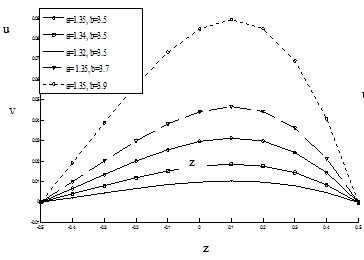Figure 3. Secondary velocity against z for $\omega$=0.01, M=2, Re=0.3, Pe=8, N=8, $G m$=4, Gr=7, h=0.5, Sc=2, $k_{p}$=0.2, m=1, t=0.1

## 4.png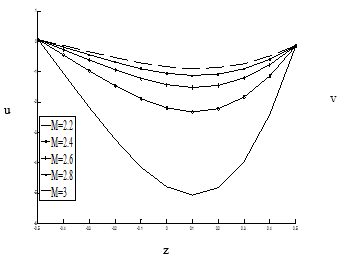Figure 4. Primary velocity against z for a=1.35, b=3.5, $\omega$=0.01, A= - 0.1, Re=0.3, Pe=8, N=8, $G m$=4, $G r$=7, h=0.5, Sc=2, $k_{p}$=0.2, m=1, t=0.1

## 5.png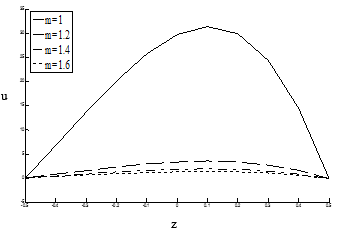Figure 5. Primary velocity against z for a=1.35, b=3.5, $\omega$=0.01, A= - 0.5, M=2, Re=0.3, Pe=8, N=8, $G_{m}$=4, $G_{r}$=7, h=0.5, Sc=2, $k_{p}$=0.2, t=0.1

## 6.png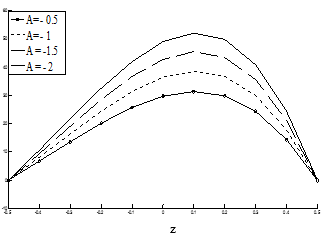Figure 6. Primary velocity against z for a=1.35, b=3.5, $\omega$=0.01, M=2, Re=0.3, Pe=8, N=8, $G_{m}$=4, $G_{r}$=7, h=0.5, Sc=2, $k_{p}$=0.2, m=1, t=0.1.

## 7.png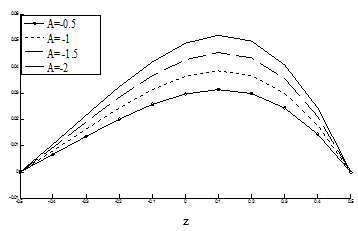Figure 7. Secondary velocity against z for $\omega$=0.01, M=2, Re=0.3, Pe=8, N=8, $G_{m}$=4, $G_{r}$=7, h=0.5, Sc=2, $k_{p}$=0.2, m=1,  t=0.1

## 8.png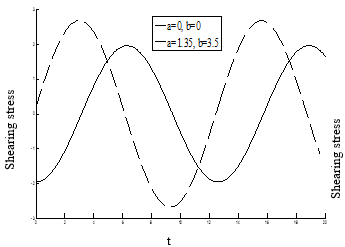Figure 8. Shearing stress due to primary flow at $z=-\frac{1}{2}$ for $\omega$=0.01, M=2, Re=0.3, Pe=8, N=8, $G_{m}$=4, $G_{r}$=7, h=0.5, Sc=2, $k_{p}$=0.2, m=1 against t

## 9.png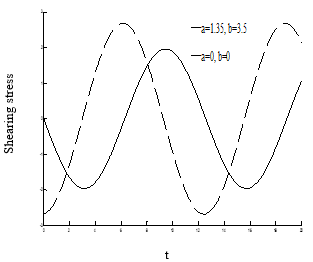Figure 9. Shearing stress due to secondary flow $z=-\frac{1}{2}$ for $\omega$=0.01, M=2, Re=0.3, Pe=8, N=8, $G_{m}$=4, $G_{r}$=7, h=0.5, Sc=2, $k_{p}$=0.2, m=1 against t

## 9.pngFigure 10. Shearing stress for primary and secondary flows at $z=-\frac{1}{2}$ for a=1.35, b=3.5, $\omega$=0.01, M=2, Re=0.3, Pe=8, N=8, $G_{m}$=4, $G_{r}$=7, h=0.5, Sc=2, $k_{p}$=0.2, m=1 against t.

## 11.png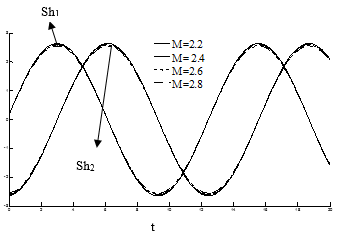Figure 11. Shearing stress for primary and secondary flows at $z=-\frac{1}{2}$ for $\omega$=0.01, a=1.35, b=3.5, Re=0.3, Pe=8, N=8, $G_{m}$=4, $G_{r}$=7, h=0.5, Sc=2, $k_{p}$=0.2, m=1 against t

## 12.png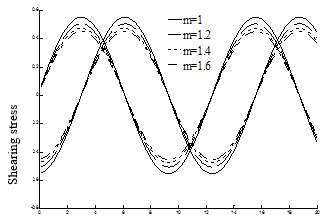Figure 12. Shearing stress for primary and secondary flows $z=-\frac{1}{2}$ for a=1.35, b=3.5, $\omega$=0.01,A=0.5, M=2, Re=0.3, Pe=8, N=8, $G_{m}$=4, $G_{r}$=7, h=0.5, Sc=2, $k_{p}$=0.2

Nomenclature

$u^{\prime} \& v^{\prime}$ are velocity of fluid $\left(\mathrm{LT}^{-1}\right), x^{\prime}, y^{\prime} \& Z^{\prime}$ are displacement variable (L), $t^{\prime}$ be time (T), $p^{\prime}$ fluid pressure (ML-1T-2), ps fluid pressure at static case (ML-1T-2), pdimensionless fluid  pressure difference, d distance between two plates (L), $T^{\prime}$ temperature of fluid (K), $C^{\prime}$ concentration of rarer component (MolL-3), Cp specific heat of fluid at constant pressure (L2T-1K-1), k thermal conducitivity (MLT-3I2), B0 magnetic field strength (MT2I-1), x, y and z are dimensionless displacement variable, u & v dimensionless velocity of fluid, t dimensionless time, T dimensionless temperature of fluid, C dimensionless concentration, Re Reynolds number, M magnetic parameter, Gr Grashof number for heat transfer, Gm Grashoff number for mass transfer, N radiation parameter, Sh dimensionless shearing stress, T0 temperature of fluid at static case (K), Tw mean temperature of fluid at z = - 0.5 (K), C0 concentration of rarer component at static case (MolL-3),Cw  mean concentration of rarer component at z= -0.5 (MolL-3), A amplitude of pressure gradient, a dimensionless relaxation time, b dimensionless retardation time, m Hall cureent parameter, k1 chemical reaction parameter (T-1), h dimensionless reaction parameter, kp dimensionless permeability parameter, D molecular diffusivity,( L2T-1), $k^{\prime}$ permeability parameter (L2), $\rho$ density of fluid, (ML-3),$\mathcal{V}$ Kinematic viscosity (L2T-1), $\sigma$ electrical conductivity (L-3M-1T3I2),  $\lambda_{1}$ relaxation time parameter  (T), $\lambda_{2}$ retardation time parameter (T), $\omega^{\prime}$ frequency of oscillation, (T-1), $\omega$ dimensionless frequency, β co-efficient of volume expansion due to heat (K-1), β* co-efficient of volume expansion due to concentration, Mol-1L3, τij viscous stress (ML-1T-2), $\eta_{0}$ dynamic viscosity (ML-1T-1), U mean flow velocity(LT-1), g acceleration due to gravity(LT-2), Pe peclet number, $\omega_{e}$ electron frequency(T-1), $\tau_{e}$ electron collision time(T).

References

 Oldroyd, J. G., “On the formulation of rheological equations of state,” Proceedings of the Royal Society of London. Series A, Mathematical and Physical Sciences, vol. 200, no. 1063, pp. 523–541, 1950. DOI: 10.1098/rspa.1950.0035.

 Oldroyd, J. G., “Non-Newtonian effects in steady motion of some idealized elastic-viscous liquids,” Proc. Roy. Soc. Lond. A, vol. 245, no. 1241, pp. 278-297, 1958. DOI: 10.1098/rspa.1958.0083.

 Dey, D., “Dusty hydromagnetic oldryod fluid flow in a horizontal channel with volume fraction and energy dissipation,” International Journal of Heat and Technology, vol. 34, no. 3, pp. 415-422, 2016. DOI: 10.18280/ijht.340310.

 Dey, D. and Khound, A. S.,  “Relaxation and retardation effects on free convective visco-elastic fluid flow past an oscillating plate,” International Journal of Computer Applications, vol. 144, no. 9, pp. 34-40, 2016. DOI: 10.5120/ijca2016910434.

 Nigam, S. D. and Singh, S. N., “Heat transfer by laminar flow between parallel plates under the action of transverse magnetic field,” Quarterly J Mechanics and Applied Mathematics, vol. 13, no. 1, pp. 85-97, 1960. DOI: 10.1093/qjmam/13.1.85.

 Soundalgekar, V. M. and Bhat, J. P., “Oscillatory channel flow and heat transfer,” Int J Pure and App Math, vol. 15, pp. 819-828, 1971.

 Soundalgekar, V. M., Vighnesam, N. V. and Takhar, H. S., “Hall and ion-slip effects in MHD Couette flow with heat transfer,” IEEE Tran Plasma Sci PS, vol. 7, no. 3, pp. 178-182, 1979. DOI: 10.1109/TPS.1979.4317226.

 Soundalgekar, V. M. and Uplekar, A. G., “Hall effects in MHD Couette flow with heat transfer,” IEEE Transactions on Plasma Science PS, vol. 14, no. 5, pp. 579-583, 1986. DOI: 10.1109/TPS.1986.4316600.

 Hossain, M. A. and Rashid, R. I. M. A., “Hall effect on hydromagnetic free convection flow along a porous flat plate with mass transfer,” J Phys Soc Japan, vol. 56, no. 1, pp. 97-104, 1987. DOI: 10.1143/JPSJ.56.97.

 Raptis, A., Massalas, C. and Tzivanidis, G., “Hydromagnetic free convection flow through a porous medium between two parallel plates,” Physics Letters A, vol. 90, no. 6, pp. 288-289, 1982. DOI: 10.1016/0375-9601(82)90118-9.

 Raptis, A. and Perdikis, C. P., “Oscillatory flow through a porous medium by the presence of free convective flow,” Int J Engng Sci, vol. 23, no. 1, pp. 51-55, 1985,  DOI: 10.1016/0020-7225(85)90015-1.

 Hassanien, I. A. and Mansour, M. A., “Unsteady magnetic flow through a porous medium between two infinite parallel plates,” Astrophysics and Space Science, vol. 163, no. 2, pp. 241-246, 1990. DOI: 10.1007/BF00655745.

 Aldoss, T. K, Al-Nimr, M. A., Jarrah, M. A., and Al-Shaer, B., “Magnetohydrodynamic mixed convection from a vertical plate embedded in a porous medium,” Numerical Heat Transfer A, vol. 28, no. 5, pp. 635-645, 1995. DOI: 10.1080/10407789508913766.

 Vajravelu, K., “Exact periodic solution of a hydromagnetic flow in a horizontal channel,” J Appl Mech, vol. 55, no. 4, pp. 981-983, 1988. DOI: 10.1115/1.3173751.

 Attia, H. A.  and Kotb, N. A., “MHD flow between two parallel plates with heat transfer,” Acta Mechanica, vol. 117, no. 1, pp. 215-220, 1996,  DOI: 10.1007/BF01181049.

 Attia, H. A., “Hall current effects on velocity and temperature fields of an unsteady Hartmann flow,” Can J Phys, vol. 76, no. 9, pp. 739-746, 1998. DOI: 10.1139/cjp-76-9-739.

 Attia, H. A.  “Unsteady Hartmann flow of a visco-elastic fluid considering the Hall effect,” Canadian J Physics, vol. 82, no. 2, pp. 127-139, 2004. DOI: 10.1139/p03-117.

 Chaudhary, R. C. and Jha, A. K., “Heat and mass transfer in elastico-viscous fluid past an impulsively started infinite vertical plate with Hall effect,” Latin American Applied Research, vol. 38, pp. 17-26, 2008.

 Rajgopal, K., Veena P. H. and Pravin V. K., “Oscillatory motion of an electrically conducting viscoelastic fluid over a stretching sheet in saturated porous medium with suction/blowing,” Mathematical Problems in Engineering, vol. 1, pp. 1-14, 2006. DOI: 10.1155/MPE/2006/60560.

 Singh, K. D. and Garg, B. P., “Radiative heat transfer in MHD oscillatory flow through porous medium bounded by two vertical porous plates,” Bull Cal Math Soc, vol. 102, pp. 129-138, 2010.

 Singh, K. D., “Viscoelastic mixed convection MHD oscillatory flow through a porous medium filled in a vertical channel,” Int J Physical and Math Sci, vol. 3, pp. 194-205, 2012.

 Singh, K. D., “Exact solution of MHD mixed convection periodic flow in a rotating vertical channel with heat radiation,” Int J Appl Mech and Engng, vol. 18, no. 3, pp. 849-869, 2013. DOI: 10.2478/ijame-2013-0051.

 Singh, K. D. and Pathak, R., “Effects of slip conditions and Hall current on an oscillatory convective flow in a rotating vertical porous channel with thermal radiation,” International Journal of Applied Mathematics and Mechanics, vol. 9, pp. 60-77, 2013.

 Seth, G. S.  Tripathi, R. and Sharma, R., “Natural convection flow past an exponentially accelerated vertical ramped temperature plate with Hall effects and heat absorption,” International Journal of Heat and Technology, vol. 33, no. 3, pp. 139-144, 2015. DOI: 10.18280/ijht.3303.

 Seth, G. S., S. Sarkar and Mahato, G. K., “Effects of Hall current on hydromagnetic free convection flow with heat and mass transfer of a heat absorbing fluid past an impulsively moving vertical plate with ramped temperature,” International Journal of Heat and Technology, vol. 31, no. 1, pp. 85-96, 2013.

 N. Ahmed, K. Sarma and H. Deka, “Soret and radiation effects on a transient mhd mass transfer flow past an infinite vertical porous plate in a rotating system with hall current,” International Journal of Heat and Technology, vol. 31, no. 1, pp. 109-116, 2013.

 Sharma, B., Sing, R. N., Gogoi, R. K. and Nath, K., “Separation of species of a binary fluid mixture confined in a channel in presence of a strong transverse magnetic field,” Hem. Ind., vol. 66, no. 2, pp. 171-180, 2012. DOI: 10.2298/HEMIND110519076S.

 Singh, K. D., Garg, B. P.  and Bansal, A. K., “Hall current effect on visco-elastic MHD oscillatory convective flow through a porous medium in a vertical channel with heat radiation,” Proc Indian Natn Sci Acad, vol. 80, no. 2, pp. 333-343, 2014.

 Cogley, A. C. L., Vincenti, W. G. and Giles, E. S., “Differential approximation for radiative heat transfer in Non-linear equations grey gas near equilibrium,” American Institute of Aeronautics and Astronautics, vol. 6, pp. 551-553, 1968.